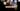# Leetcode | Solution of Missing Number in JavaScript

March 25th, 2020
|

In this post, we will solve the problem missing number from leetcode and compute the time and space complexities. Let's begin.

# Problem Statement

The question can be found at leetcode missing number problem.

The problem states that we need to determine a missing number from a series `0,1,2,3,4,...n` of length `n`.

# Solution

Before discussing a solution let's agree on the fact that a series `0,1,2,3,...n` has length `n+1` (numbers from 1 to n and a zero), so if we are given a series of length `n`, a number from the series is missing for sure.

Agreed? Good...let's proceed.

The approach is as follows

• First, we'll calculate the sum of all numbers in a series of length `n+1`
• Next, we'll subtract all the numbers in the given series from the calculated sum
• The remaining number after we subtract all the number from the input is the missing number

We have discussed the approach, I urge you to go ahead on leetcode and give it another try.If you are here, it means something went wrong in implementation or you are just too lazy. In any case, let's see a simple implementation of the above logic.
``````var missingNumber = function (nums) {
const length = nums.length;
let sum = ((length + 1) * length) / 2;

for (let i = 0; i < length; i++) {
sum = sum - nums[i];
}

return sum;
};``````

The code is here is pretty simple, the only thing to note is, on line 3, we calculated sum using a famous formula instead of looping through all numbers. It saves us some time in terms of computation.

After submission, here's the result

``````Status: Accepted
Runtime: 52ms
Memory: 36MB``````

## Time and space complexity

### Time complexity

We are looping over the num array once, so the time complexity is O(n).

### Space complexity

We are using extra space only to keep for some variables, thus, space complexity would be O(1).

# Summary

So, we solved the missing number problem by using a simple mathematical formula and a for loop. Finally, calculated the time and space complexities.

I hope you enjoyed solving this question. This is it for this one, complete source code for this post can be found on my Github Repo. Will see you in the next one.